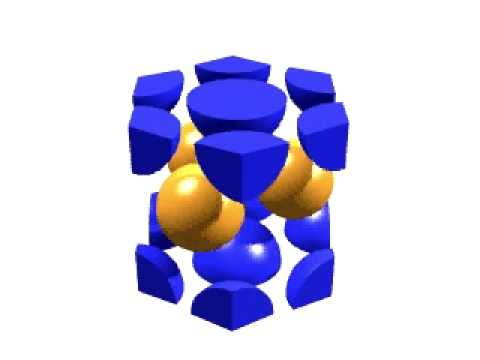# Question #17dc3

Sep 2, 2017

The compound is $\text{A"_2"B"_3}$.

#### Explanation:

This is an ionic compound. Because the anions are larger, they form the HCP array and the cations occupy the tetrahedral holes.

Hexagonal closest packing

Below is a diagram of an HCP unit cell.There is $\frac{1}{6}$ atom at each corner, $\frac{1}{2}$ atom at the centre of each hexagonal face, and 3 atoms inside the cell.

$\text{Number of atoms" = 12 color(red)(cancel(color(black)("corners"))) × (1/6 "atom")/(1 color(red)(cancel(color(black)("corner")))) + 2 color(red)(cancel(color(black)("faces"))) × (1/2 "atom")/(1 color(red)(cancel(color(black)("face")))) + "3 atoms" = "(2 + 1 + 3) atoms" = "6 atoms}$

Tetrahedral holes

A tetrahedral hole is the space between four atoms that are touching each other.How many tetrahedral holes in an HCP unit cell?

For simplicity, let's examine just the parallepiped that makes up one-third of the unit cell.Since this is one-third of a unit cell, it contains two atoms.(From SlidePlayer)

There are four tetrahedral holes — or two for each atom.

The unit cell contains 6 atoms, so it contains 12 tetrahedral holes.

Formula of the compound

The unit cell contains 6 anions of $\text{B}$ and 12 tetrahedral holes.

One-third of the holes contain cations of $\text{A}$ (four cations).

Hence, the formula of the unit cell is ${\text{A"_4"B}}_{6}$.

The empirical formula of the compound is ${\text{A"_2"B}}_{3}$.